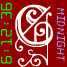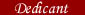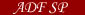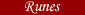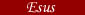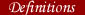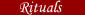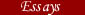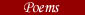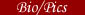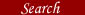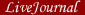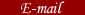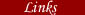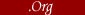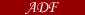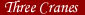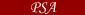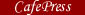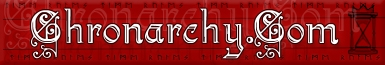# Wing Formations

### from the Greek Magical Papyri

-Michael J Dangler

Wing formations: These formations are generally made from magical words, and often show up as pyramids next to each other. Most often, you drop a letter off at each new line.

```A
E E
E E E
I I I I
O O O O O
Y Y Y Y Y Y
O O O O O O O

O O O O O O O
Y Y Y Y Y Y
O O O O O
I I I I
E E E
E E
A```

-PGM I.15

```A
B A
R B A
A R B A
N A R B A
A N A R B A
K A N A R B A
A K A N A R B A
R A K A N A R B A
K R A K A N A R B A
A K R A K A N A R B A

A K R A K A N A R B A
K R A K A N A R B A
R A K A N A R B A
A K A N A R B A
K A N A R B A
A N A R B A
N A R B A
A R B A
R B A
B A
A```

-PGM II.1; II.67

```A
L A
A L A
L A L A
A L A L A
T A L A L A
N T A L A L A
A N T A L A L A
S A N T A L A L A

S A N T A L A L A
A N T A L A L A
N T A L A L A
T A L A L A
A L A L A
L A L A
A L A
L A
A```

-PGM II.5; II.67

Content © 2003 - 2004, Michael J Dangler
Updated on 09/30/2004. Site Credits / Email Me!